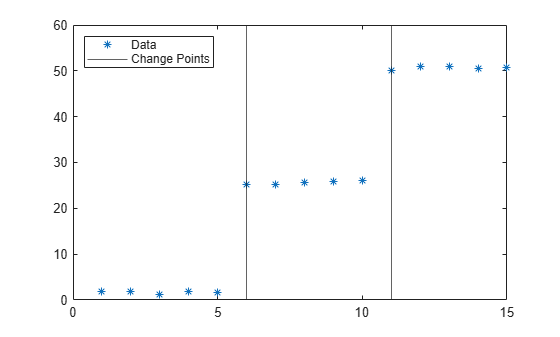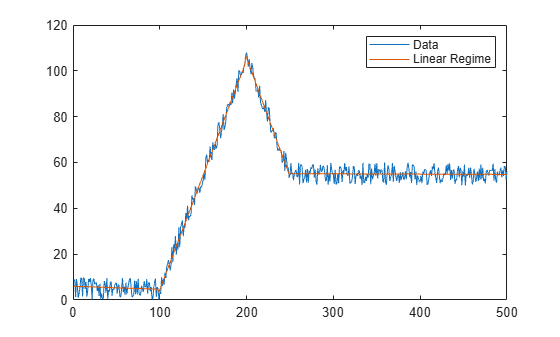ischange

Find abrupt changes in data

Description

example

TF = ischange(A) returns a logical array whose elements are 1 (true) when there is an abrupt change in the mean of the corresponding elements of A.

example

TF = ischange(A,method) specifies how to define a change point in the data. For example, ischange(A,'variance') finds abrupt changes in the variance of the elements of A.

example

TF = ischange(___,dim) specifies the dimension of A to operate along for either of the previous syntaxes. For example, ischange(A,2) computes change points for each row of a matrix A.

example

TF = ischange(___,Name,Value) specifies additional parameters for finding change points using one or more name-value pair arguments. For example, ischange(A,'MaxNumChanges',m) detects no more than m change points.

example

[TF,S1] = ischange(___) also returns information about the line segments in between change points. For example, [TF,S1] = ischange(A) returns a vector S1 containing the mean of data between change points of a vector A.

example

[TF,S1,S2] = ischange(___) returns additional information about the line segments in between change points. For example, [TF,S1,S2] = ischange(A) returns a vector S1 that contains the mean for each segment, as well as a vector S2 that contains the variance for each segment of a vector A.

Examples

collapse all

Create a vector of noisy data, and compute the abrupt changes in the mean of the data.

A = [ones(1,5) 25*ones(1,5) 50*ones(1,5)] + rand(1,15);
TF = ischange(A)
TF = 1x15 logical array

0   0   0   0   0   1   0   0   0   0   1   0   0   0   0

To compute the mean of the data in between change points, specify a second output argument.

[TF,S1] = ischange(A);
plot(A,'*')
hold on
stairs(S1)
legend('Data','Segment Mean','Location','NW')Create a vector of noisy data, and compute abrupt changes in the slope and intercept of the data. Setting a large detection threshold reduces the number of change points detected due to noise.

A = [zeros(1,100) 1:100 99:-1:50  50*ones(1,250)] + 10*rand(1,500);
[TF,S1,S2] = ischange(A,'linear','Threshold',200);
segline = S1.*(1:500) + S2;
plot(1:500,A,1:500,segline)
legend('Data','Linear Regime')As an alternative to providing a threshold value, you also can specify the maximum number of change points to detect.

[TF,S1,S2] = ischange(A,'linear','MaxNumChanges',3);

Compute abrupt changes in the mean for each row of a matrix.

A = diag(25*ones(5,1)) + rand(5,5)
A = 5×5

25.8147    0.0975    0.1576    0.1419    0.6557
0.9058   25.2785    0.9706    0.4218    0.0357
0.1270    0.5469   25.9572    0.9157    0.8491
0.9134    0.9575    0.4854   25.7922    0.9340
0.6324    0.9649    0.8003    0.9595   25.6787

TF = ischange(A,2)
TF = 5x5 logical array

0   1   0   0   0
0   1   1   0   0
0   0   1   1   0
0   0   0   1   1
0   0   0   0   1

Input Arguments

collapse all

Input data, specified as a vector, matrix, multidimensional array, table, or timetable.

Data Types: single | double | table | timetable

Change detection method, specified as one of the following:

• 'mean' — Find abrupt changes in the mean of the data.

• 'variance' — Find abrupt changes in the variance of the data.

• 'linear' — Find abrupt changes in the slope and intercept of the data.

Operating dimension, specified as a positive integer scalar. By default, ischange operates along the first dimension whose size does not equal 1.

For example, if A is a matrix, then ischange(A,1) operates along the rows of A, computing change points for each column.ischange(A,2) operates along the columns of A, computing change points for each row.Data Types: single | double | int8 | int16 | int32 | int64 | uint8 | uint16 | uint32 | uint64

Name-Value Arguments

Specify optional comma-separated pairs of Name,Value arguments. Name is the argument name and Value is the corresponding value. Name must appear inside quotes. You can specify several name and value pair arguments in any order as Name1,Value1,...,NameN,ValueN.

Example: TF = ischange(A,'MaxNumChanges',5)
Data Options

collapse all

Sample points, specified as the comma-separated pair consisting of 'SamplePoints' and either a vector of sample point values or one of the options in the following table when the input data is a table. The sample points represent the x-axis locations of the data, and must be sorted and contain unique elements. Sample points do not need to be uniformly sampled. The vector [1 2 3 ...] is the default.

When the input data is a table, you can specify the sample points as a table variable using one of the following options.

Option for Table InputDescriptionExamples
Variable name

A character vector or scalar string specifying a single table variable name

'Var1'

"Var1"

Scalar variable index

A scalar table variable index

3

Logical vector

A logical vector whose elements each correspond to a table variable, where true specifies the corresponding variable as the sample points, and all other elements are false

[true false false]

Function handle

A function handle that takes a table variable as input and returns a logical scalar, which must be true for only one table variable

@isnumeric

vartype subscript

A table subscript generated by the vartype function that returns a subscript for only one variable

vartype('numeric')

Note

This name-value pair is not supported when the input data is a timetable. Timetables always use the vector of row times as the sample points. To use different sample points, you must edit the timetable so that the row times contain the desired sample points.

Example: ischange([1 2 3 4 5 6],'linear','SamplePoints',[1 2 3 10 20 30])

Example: ischange(T,'linear','SamplePoints',"Var1")

Data Types: single | double | datetime | duration

Table variables to operate on, specified as the comma-separated pair consisting of 'DataVariables' and one of the options in this table. The 'DataVariables' value indicates which variables of the input table to examine for change points. The data type associated with the indicated variables must be double or single. Other variables in the table not specified by 'DataVariables' are not operated on, so the output contains false values for those variables.

OptionDescriptionExamples
Variable name

A character vector or scalar string specifying a single table variable name

'Var1'

"Var1"

Vector of variable names

A cell array of character vectors or string array where each element is a table variable name

{'Var1' 'Var2'}

["Var1" "Var2"]

Scalar or vector of variable indices

A scalar or vector of table variable indices

1

[1 3 5]

Logical vector

A logical vector whose elements each correspond to a table variable, where true includes the corresponding variable and false excludes it

[true false true]

Function handle

A function handle that takes a table variable as input and returns a logical scalar

@isnumeric

vartype subscript

A table subscript generated by the vartype function

vartype('numeric')

Example: ischange(T,'DataVariables',["Var1" "Var2" "Var4"])

Change Point Options

collapse all

Change point threshold, specified as the comma-separated pair consisting of 'Threshold' and a nonnegative scalar. Increasing the threshold greater than 1 produces fewer change points.

The threshold value defines the number of detected change points and cannot be specified when 'MaxNumChanges' is specified.

Data Types: double | single | int8 | int16 | int32 | int64 | uint8 | uint16 | uint32 | uint64

Maximum number of change points to detect, specified as the comma-separated pair consisting of 'MaxNumChanges' and a positive integer scalar. ischange uses an automatic threshold that computes no more than the specified value of change points, thus 'Threshold' cannot be specified when 'MaxNumChanges' is specified.

Data Types: double | single | int8 | int16 | int32 | int64 | uint8 | uint16 | uint32 | uint64

Output Arguments

collapse all

Change point indicator, returned as a vector, matrix, or multidimensional array. TF is the same size as A.

Data Types: logical

Mean or slope of data between change points, returned as a vector, matrix, multidimensional array, table, or timetable.

• If the change point detection method is 'mean' or 'variance', then S1 contains the mean for each segment.

• If the method is 'linear', then S1 contains the slope for each segment.

s1 has the same type is the input data.

Data Types: double | single | table | timetable

Variance or intercept of data between change points, returned as a vector, matrix, multidimensional array, table, or timetable.

• If the change point detection method is 'mean' or 'variance', then S2 contains the variance for each segment.

• If the method is 'linear', then S2 contains the intercept for each segment.

s2 has the same type is the input data.

Data Types: double | single | table | timetable

collapse all

Change Points

A vector of data A contains a change point if it can be split into two segments A1 and A2 such that

$C\left({A}_{1}\right)+C\left({A}_{2}\right)+\tau

$\tau$ is the threshold value specified by the 'Threshold' parameter, and C represents a cost function.

For example, the cost function for detecting abrupt changes in the mean is $C\left(x\right)=N\mathrm{var}\left(x\right)$, where N is the number of elements in a vector x. The cost function measures how well a segment is approximated by its mean.

ischange iteratively minimizes the sum of the cost functions to determine the number of change points k and their locations such that

$C\left({A}_{1}\right)+C\left({A}_{2}\right)+...+C\left({A}_{k}\right)+k\tau

 Killick R., P. Fearnhead, and I.A. Eckley. "Optimal detection of changepoints with a linear computational cost." Journal of the American Statistical Association. Vol. 107, Number 500, 2012, pp.1590-1598.